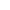# 口算是影响小学生数学学习最大的问题！（附速算技巧）

2021-07-21“粗心、粗心，又是粗心？你看，连9+3都算错，难道不是粗心吗？”这是粗心吗？其实这是口算没有过关。

1、审题不清

2、容易被假想迷惑

3、多受负迁移的影响

1.多做多练，熟能生巧

“冰冻三尺，非一日之寒”，口算能力是孩子必备的基本功，我们应作出长计划，短安排，有目的、有计划、有步骤地进行教学和训练，体现出循序渐进的基本原则和按新的课程标准进行教学。

2.保质、保量的训练

3.训练形式多样化

4.理解算理，掌握巧算的方法

5.养成良好的计算习惯1、十几乘十几：

1×1=1

2＋4＝6

2×4＝8

12×14=168

2、头相同，尾互补(尾相加等于10)：

2＋1＝3

2×3＝6

3×7＝21

23×27=621

3、第一个乘数互补，另一个乘数数字相同：

3+1=4

4×4=16

7×4=28

37×44=1628

4、几十一乘几十一：

2×4=8

2+4=6

1×1=1

21×41=861

5、11乘任意数：

2+3=5

3+1=4

1+2=3

2+5=7

2和5分别在首尾

11×23125=254375

6、十几乘任意数：

13个位是3

3×3+2=11

3×2+6=12

3×6=18

13×326=4238